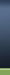HOME BZU Mail Box Online Games Radio and TV Cricket All Albums

#1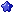bonfire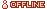M.Arsalan Qureshi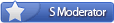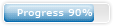Join Date: Oct 2008 Location: Garden Town, Multan Cantt Posts: 616 Program / Discipline: BSTS Class Roll Number: 09-31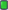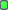Chapter 11: Op-Amp Applications

Chapter 11: Op-Amp Applications

One of the most common op-amp circuits is the inverting constant-gain multiplier, which provides a precise gain or amplification. Figure 11.1 shows a standard circuit connection, with the resulting gain being given by(11.1)FIG. 11.1
Fixed-gain amplifier. EXAMPLE 11.1 Determine the output voltage for the circuit of Fig. 11.2 with a sinusoidal input of 2.5 mV.FIG. 11.2
Circuit for Example 11.2.
Solution: The circuit of Fig. 11.2 uses a 741 op-amp to provide a constant or fixed gain, calculated from Eq. (11.1) to beThe output voltage is thenA noninverting constant-gain multiplier is provided by the circuit of Fig. 11.3, with the gain given by(11.2)FIG. 11.3
Noninverting fixed-gain amplifier.
EXAMPLE 11.2 Calculate the output voltage from the circuit of Fig. 11.4 for an input of 120V. Solution: The gain of the op-amp circuit is calculated using Eq. (11.2) to beThe output voltage is thenFIG. 11.4
Circuit for Example 11.2.
Multiple-Stage Gains
When a number of stages are connected in series, the overall gain is the product of the individual stage gains. Figure 11.5 shows a connection of three stages. The first stage is connected to provide noninverting gain as given by Eq. (11.1). The next two stages provide an inverting gain given by Eq. (11.1). The overall circuit gain is then noninverting and is calculated bywhereFIG. 11.5
Constant-gain connection with multiple stages.
EXAMPLE 11.3 Calculate the output voltage using the circuit of Fig. 11.5 for resistor components of value Rf = 470 k, R1 = 4.3 k, R2 = 33 k, and R3 = 33 kfor an input of 80V. Solution: The amplifier gain is calculated to beso thatEXAMPLE 11.4 Show the connection of an LM124 quad op-amp as a three-stage amplifier with gains of +10, –18, and –27. Use a 270-kfeedback resistor for all three circuits. What output voltage will result for an input of 150V?
Solution: For the gain of +10,For the gain of –18,For the gain of –27,The circuit showing the pin connections and all components used is given in Fig. 11.6. For an input of V1 = 150V, the output voltage is
Vo = A1A2A3V1 = (10)(–18)(–27)(150V) = 4860 (150V) = 0.729 VFIG. 11.6
Circuit for Example 11.4 (using LM124).
A number of op-amp stages could also be used to provide separate gains, as demonstrated in the next example. EXAMPLE 11.5 Show the connection of three op-amp stages using an LM348 IC to provide outputs that are 10, 20, and 50 times larger than the input. Use a feedback resistor of Rf = 500 kin all stages.
Solution: The resistor component for each stage is calculated to beThe resulting circuit is drawn in Fig. 11.7.FIG. 11.7
Circuit for Example 11.5 (using LM348).
__________________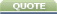Tags applications, chapter, opampCurrently Active Users Viewing This Thread: 1 (0 members and 1 guests)
 Thread Tools Search this ThreadShow Printable VersionEmail this Page Search this Thread: Advanced Search Display Modes Rate This ThreadLinear ModeSwitch to Hybrid ModeSwitch to Threaded Mode Rate This Thread: 5 : Excellent 4 : Good 3 : Average 2 : Bad 1 : Terrible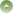Posting Rules You may not post new threads You may not post replies You may not post attachments You may not edit your posts BB code is On Smilies are On [IMG] code is On HTML code is OffTrackbacks are On Pingbacks are On Refbacks are On Forum Rules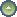Similar Threads Thread Thread Starter Forum Replies Last Post bonfire Discrete Mathematics 0 14-04-2011 07:13 PM bonfire Discrete Mathematics 0 14-04-2011 07:08 PM bonfire Discrete Mathematics 0 14-04-2011 07:03 PM bonfire Electrical Circuits 0 19-03-2011 08:01 PM bonfire Computer Networks 0 17-02-2011 06:32 PM

Best view in Firefox
Almuslimeen.info | BZU Multan | Dedicated server hosting
Note: All trademarks and copyrights held by respective owners. We will take action against any copyright violation if it is proved to us.

All times are GMT +5. The time now is 11:47 PM.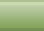Contact Us - BZU Multan - Archive - Top